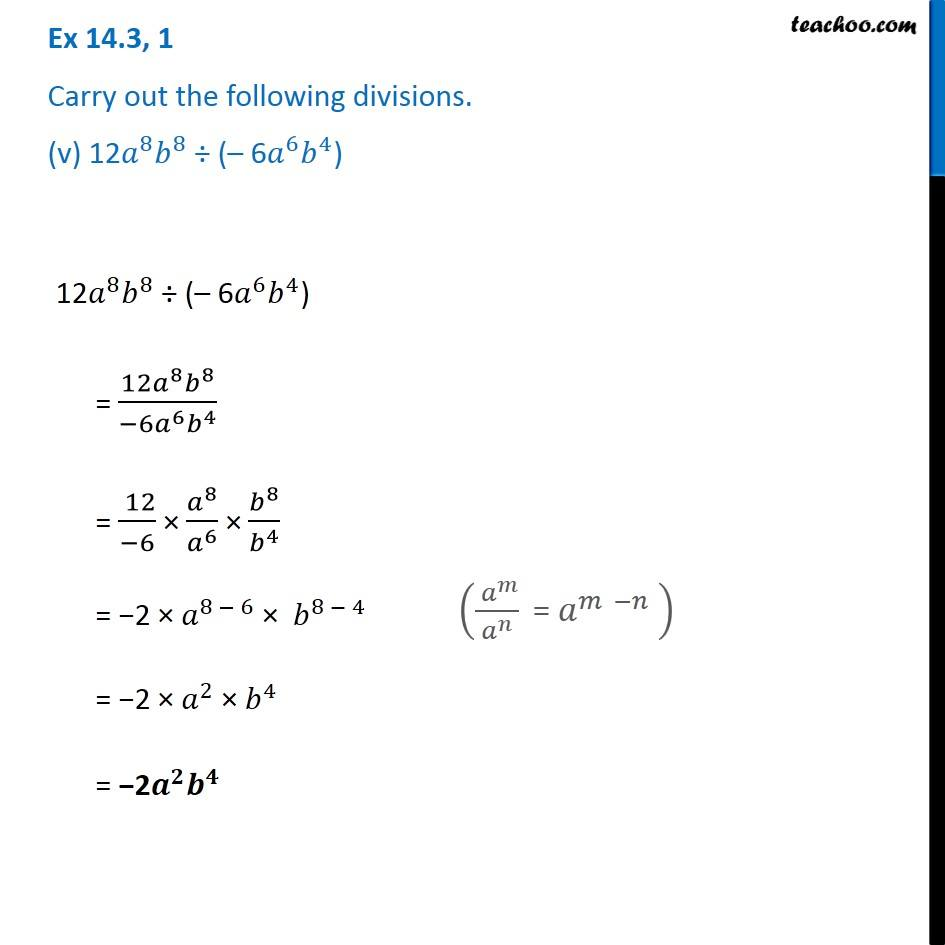1. Chapter 14 Class 8 Factorisation
2. Serial order wise
3. Ex 14.3

Transcript

Ex 14.3, 1 Carry out the following divisions. (v) 12𝑎^8 𝑏^8 ÷ (– 6𝑎^6 𝑏^4) 12𝑎^8 𝑏^8 ÷ (– 6𝑎^6 𝑏^4) = (12𝑎^8 𝑏^8)/(−6𝑎^6 𝑏^4 ) = ( 12)/(−6) × 𝑎^8/𝑎^6 × 𝑏^8/𝑏^4 = −2 × 𝑎^(8 − 6) × 𝑏^(8 − 4) = −2 × 𝑎^2 × 𝑏^4 = −2𝒂^𝟐 𝒃^𝟒 (( 𝑎^𝑚)/𝑎^𝑛 " = " 𝑎^(𝑚 −𝑛) " " )

Ex 14.3

Chapter 14 Class 8 Factorisation
Serial order wise